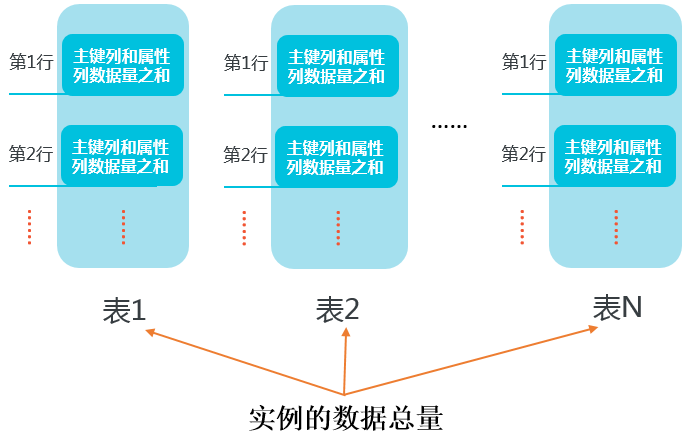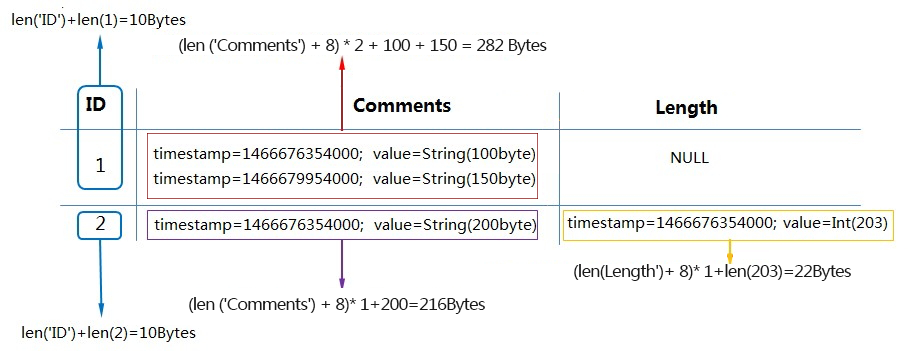## 计算行数据量

• 主键列的数据量=主键列的名字长度之和+主键列的值的数据量之和

• 属性列的数据量计算方式，请参考本文档中关于行及表的数据量计算示例的具体说明。

String UTF-8字符串占用的字节数（表格存储允许值为空的String类型，如果字符串为空，则数据大小为0。）
Integer 8
Double 8
Boolean 1
Binary 二进制数据占用的字节数

1 timestamp=1466676354000,value=‘zhangsan’ timestamp=1466676354000,value=20

timestamp=1466676354000,value=String（100 Bytes）

timestamp=1466679954000,value=String（150 Bytes）

• 当MaxVersions=2，TTL=2592000时：
单个属性列的数据量=（属性列名字长度之和+8）*有效版本个数+该属性列所有有效版本的值数据量之和
说明 在使用多版本（即Max versions>1）或者使用了`TTL（即TTL=-1）`的情况下，每个版本号需要占用8字节（以下提到的timestamp等同于版本号）。
该行数据量=10+20+22+282=334 Bytes，详情如下：
• 主键列数据量=len(‘ID’)+len(1)=10 Bytes
• 属性列Name数据量=(len(‘Name’)+8)*1+len(‘zhangsan’)=20 Bytes
• 属性列Length数据量=(len(‘Length’)+8)*1+len(20)=22 Bytes
• 属性列Comments数据量=`(len(‘Comments’)+8)*2+100+150=282 Bytes`
• 当MaxVersions=1，TTL=-1时：

单个属性列的数据量=属性列名字长度之和+属性列的值的数据量之和

说明
• 在不使用多版本（即Max versions=1）且不使用TTL（即TTL=-1）的场景下，版本号不占用字节。

该行数据量=10+12+14+158=194 Bytes，详情如下：

• 主键列数据量=`len(‘ID’)+len(1)=10 Bytes`
• 属性列Name数据量=`len(‘Name’)+len(‘zhangsan’)=12 Bytes`
• 属性列Length数据量=`len(‘Length’)+len(20)=14 Bytes`

## 计算表数据量